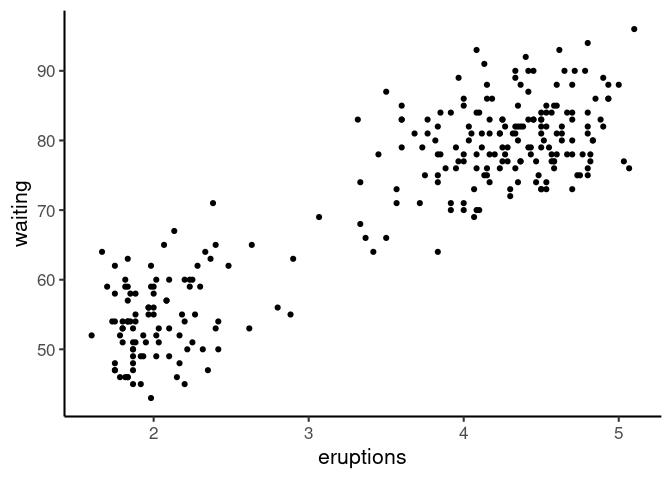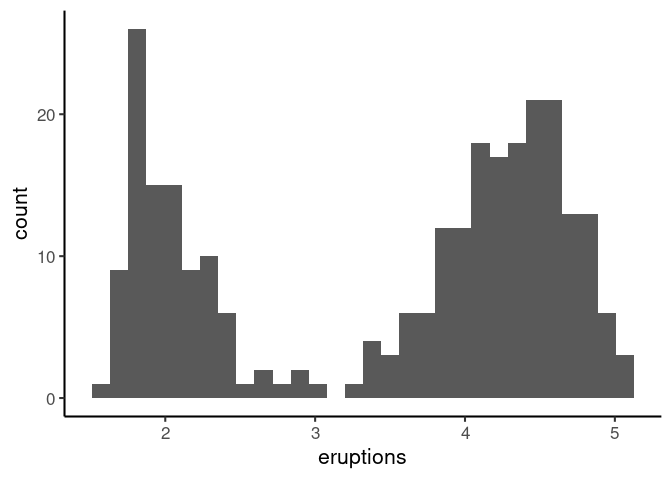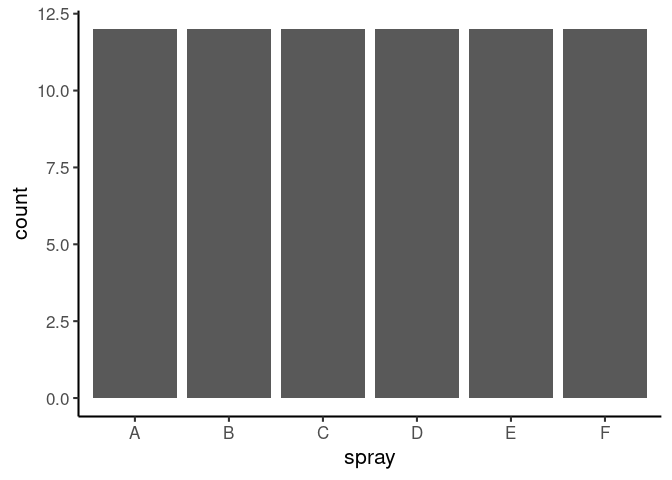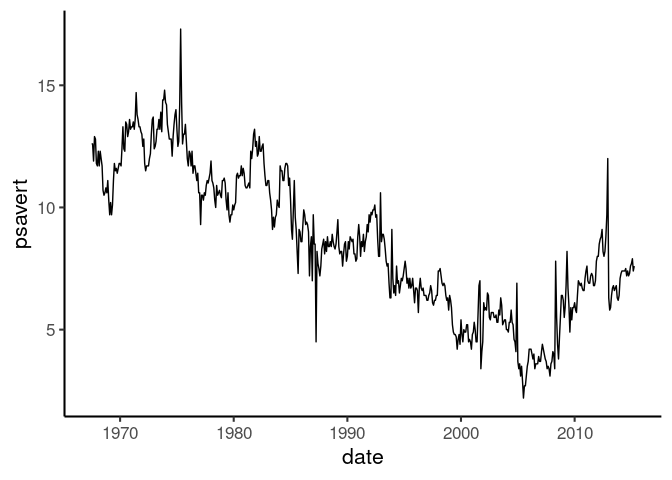# Three key ingredients to make a data graphic

Tuesday, Jul 6, 2021
Data Graphics R

When constructing a data graphics using grammer of graphics, it’s worth noting there there are three key ingredients:

1. Data: Can’t do much of a data graphics without data.
2. Axis Mapping: Pick one or two variables from data and map those to axes (x, y or both x & y).
3. Geometrical Element: Select an appropriate geometrical element to visually display the data.

Thinking in this way give us a good starting point to build common plots but more importantly, it provides a flexible framework to construct the plots we want. This is what I slowly will attempt to learn.

Let’s see how these elements come together using some examples:

### Scatter Plot

For scatter plot, we need two numerical variables.

• Data: Old faithful
• Axis: x = eruptions, y = waiting
• Geometrical Element: point
ggplot(faithful, aes(eruptions, waiting)) +
geom_point()### Histogram

For histogram, we need one numerical variable.

• Data: Old faithful
• Axis: x = eruptions
• Geometrical Element: histogram
ggplot(faithful, aes(eruptions)) +
geom_histogram()### Bar chart

Bar chart has one categorical variable and the each bar’s height represents the count for each category.

• Data: InsectSprays
• Axis: x = spray
• Geometrical Element: bar
ggplot(InsectSprays, aes(spray)) +
geom_bar()### Time series

Time series is a plot of numeric variable as a function of time.

• Data: ggplot2::economics
• Axis: x = date, y = psavert (personal savings rate)
• Geometrical Element: line
ggplot(economics, aes(date, psavert)) +
geom_line()